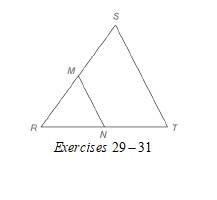Chapter 4.2, Problem 30E### Elementary Geometry for College St...

6th Edition
Daniel C. Alexander + 1 other
ISBN: 9781285195698

#### Solutions

Chapter
Section### Elementary Geometry for College St...

6th Edition
Daniel C. Alexander + 1 other
ISBN: 9781285195698
Textbook Problem
174 views

# In Exercises 29 to 31, M and N are the midpoints of sides R S ¯ and R T ¯ of ∆ R S T , respectively. Given: M N = x 2 + 5   S T = x ( 2 x + 5 ) Find: x , M N , S TTo determine

To find:

x, MN, and ST

For MN=x2+5

ST=x(2x+5)

Explanation

Calculation:

Given,

MN=x2+5

ST=x(2x+5)

M and N are the midpoints of sides RS¯ and RT¯ of RST, respectively.

A line segment that joins the midpoints of two sides of a triangle is parallel to the third side and has a length equal to one-half the length of the third side.

Thus,

MN=12ST

x2+5=12·x(2x+5)

Multiply; by 2 on both sides,

2(x2+5)=2·12·x(2x+5)

2(x2+5)=x(2x+5)

Apply the distributive property of multiplication over addition to the left and right of the above equation

### Still sussing out bartleby?

Check out a sample textbook solution.

See a sample solution

#### The Solution to Your Study Problems

Bartleby provides explanations to thousands of textbook problems written by our experts, many with advanced degrees!

Get Started

#### Find more solutions based on key concepts